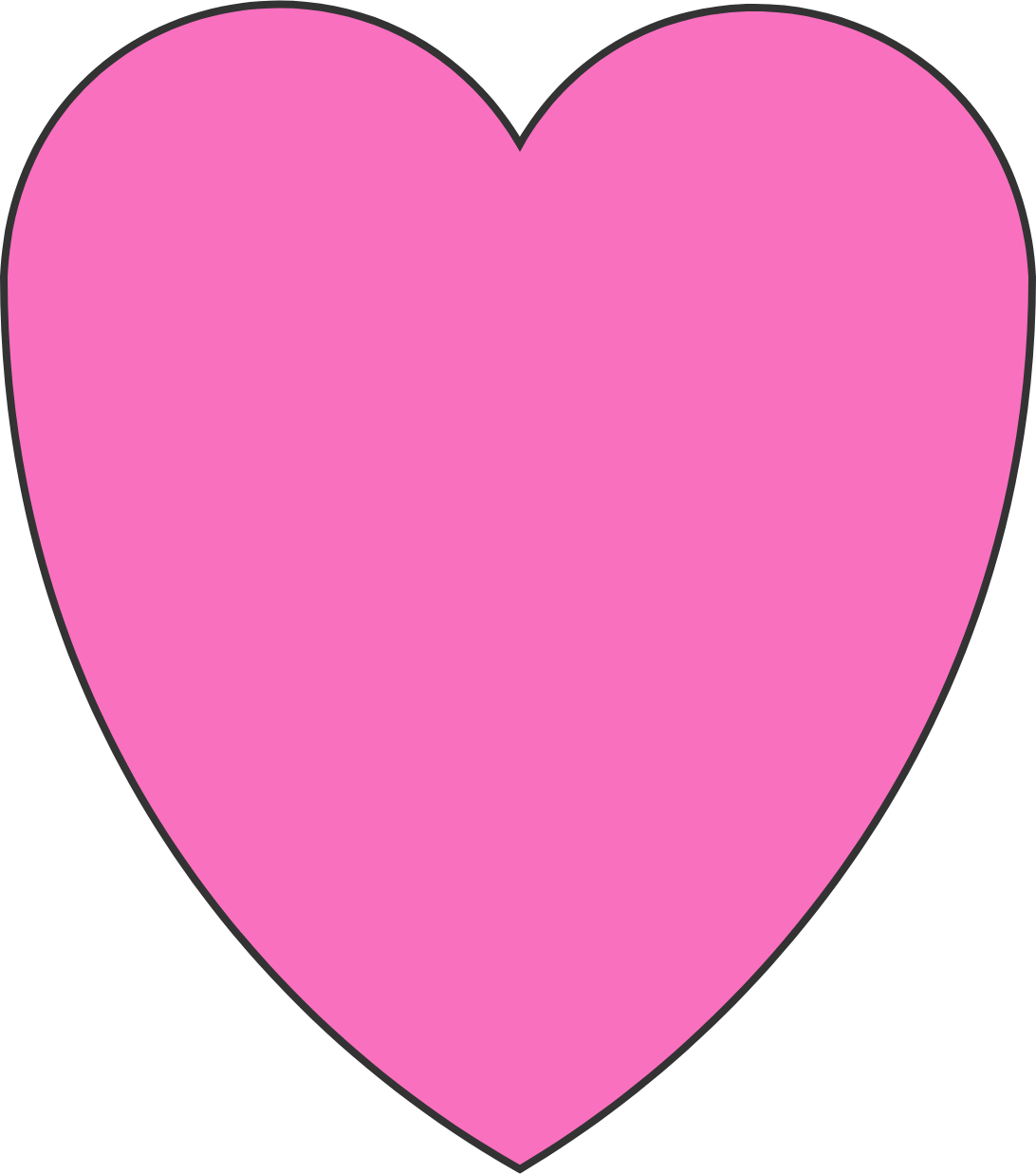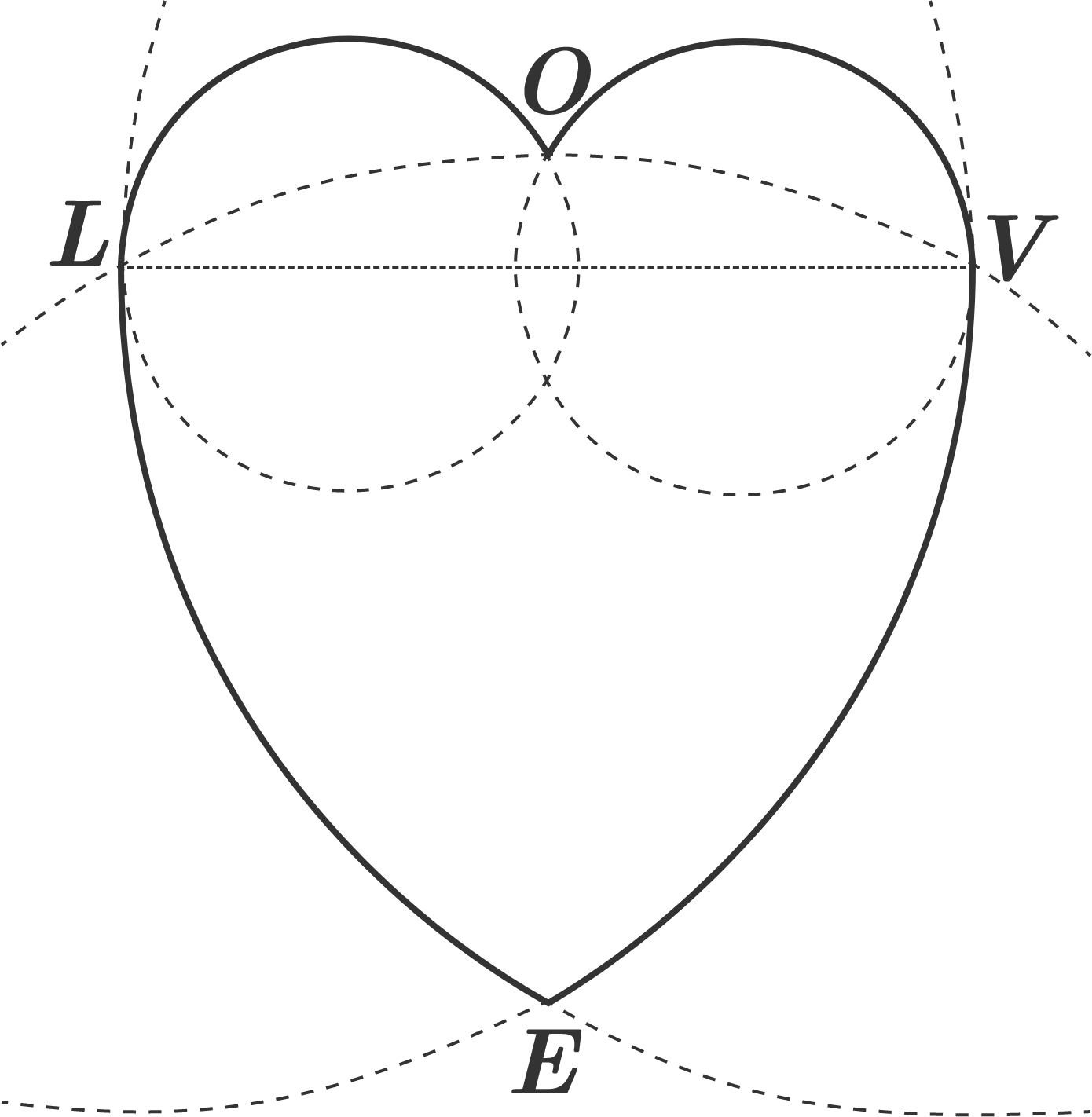# My, My! What a Pretty Valentine Pool We Have Here!After planning a cake, Mai Valentine is planning to build a heart-shaped swimming pool--like the one above--for Valentine's Day celebrations where a lot of her friends will attend. But she wants the pool to have some mathematical meaning and asks Joey Wheeler, one of the friends who is also an active Brilliant user, for help.

Joey gladly suggests an idea: add double caps like $\frown\frown$ on top of a Reuleaux triangle:

• The "double caps" are part of 2 small, identical, intersecting circles, which are the arcs $\overparen{\small{LO}} \overparen{\small{OV}}$ in the diagram below.
• The Reuleaux triangle $\big($the curved triangle $ELV\big)$ is the intersection of 3 large circles centered at $E, L, V,$ respectively.More specifically, as shown above, the 2 small circles intersect at point $O$ lying on the circle centered at $E,$ and are each tangent to one of the other two large circles at points $L$ and $V,$ respectively.

If $|LV| = 1$ decameter (10 meters), what is the total area of the bolded Valentine swimming pool $LOVE$ in squared decameters $\big($in 100 $\text{m}^2\big)?$